#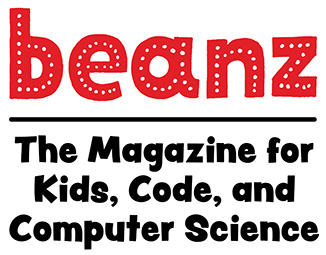beanz Magazine

## Binary Numbers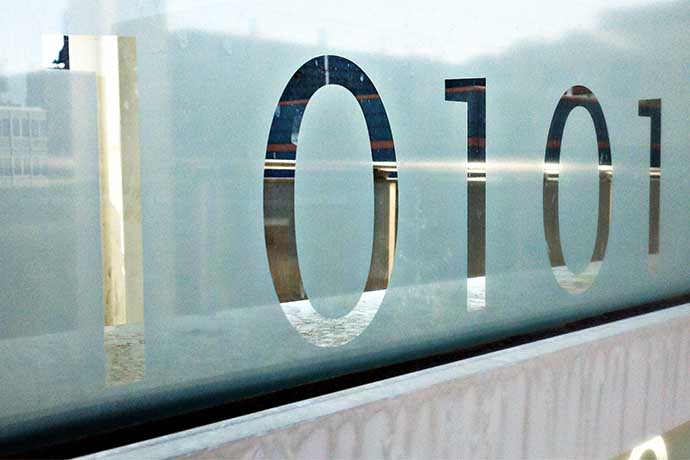Chris McClanahan on Flickr

This project teaches you about binary numbers and how to translate them to decimal numbers we recognize.

When you see a letter T on your computer screen, did you know the computer doesn’t actually use the letter T? Instead, it uses a string of numbers, for example, 01010100. This is called a binary number because it uses two numbers, 0 and 1.

Computers transport, calculate, and translate binary numbers because computer hardware circuits only have two electrical states, on or off. These two states can be represented as zero (off) or one (on). All letters of the alphabet, numbers, and symbols are converted to eight (or more) character binary numbers as you work with them in software on your computer.

However, you can have fun with binary numbers without diving deeply into how computers translate binary numbers into letters, numbers, and characters.

### How Binary Numbers Work

Here’s how simple binary numbers work. First, each position in a string of ones and zeroes represents a power of 2. A zero means no value is counted. A one means the power of 2 is added to your total number. The value of the power of 2 depends on the position of the one in the string. You calculate or decode a binary number by adding up the power of 2 values, working from right to left. Here’s an example how this works for binary number 110001011:

 1 1 0 0 0 1 0 1 1 Write a binary number 1 or 0 in each cell 256 128 64 32 16 8 4 2 1 Position Value (as a power of 2) 256 128 8 2 1 Write value of each 1 then write total here: 256 + 128 + 8 + 2 + 1 = 395

 0 0 Write a binary number 1 or 0 in each cell 2 1 Position Value (as a power of 2) 0 0 Write value of each 1 then write total here: 0 + 0 = 0

The two-digit binary number 1 1 has a value of 3. The left 1 is in the position with a power of 2 value of 2 while the right 1 is in the position with a value of 1.

 0 0 Write a binary number 1 or 0 in each cell 2 1 Position Value (as a power of 2) 2 1 Write value of each 1 then write total here: 2 + 1 = 3

The three-digit binary number 1 1 0 equals 6 (4 + 2):

 1 1 0 Write a binary number 1 or 0 in each cell 4 2 1 Position Value (as a power of 2) 4 2 Write value of each 1 then write total here: 4 + 2 = 6

If you want to play further with binary numbers, the tables below show the power of 2 values for binary numbers up to nine digits. At the bottom of this article is a link to an Excel worksheet to help convert binary numbers to decimal numbers, as well as a blank worksheet (PDF) to play around converting decimal numbers to binary and back again.

Here’s a secret: add up the power of 2 values to find the maximum number you can represent with your binary number string. For example, a two-digit binary number string has possible power of 2 values of 2 and 1. So 3 is the highest number you can translate into a two-digit binary number. A six-digit binary number can only represent numbers up to 63 (32 + 16 + 8 + 4 + 2 + 1).

How many numbers can you translate into binary numbers? Can you write a random binary number and translate it into a decimal number we recognize? For more fun, figure out the highest number that can be represented with a ten-digit binary number.

### Can You Translate/Decode These Binary Numbers?

Print out and use the blank tables below to translate/decode these binary numbers, or any numbers with 1 and 0s.

0 1
1 0 1
0 1 1 0
1 1 0 0 1
0 1 1 0 0 0
1 1 1 0 1 0 0
1 0 0 1 1 0 0 0 0

 Write a binary number 1 or 0 in each cell 2 1 Position Value (as a power of 2) Write value of each 1 then write total here:
 Write a binary number 1 or 0 in each cell 4 2 1 Position Value (as a power of 2) Write value of each 1 then write total here:
 Write a binary number 1 or 0 in each cell 8 4 2 1 Position Value (as a power of 2) Write value of each 1 then write total here:
 Write a binary number 1 or 0 in each cell 256 128 64 32 16 8 4 2 1 Position Value (as a power of 2) Write value of each 1 then write total here:

#### Binary Number Worksheet (Excel format)

https://www.kidscodecs.com/binary-numbers-converter

#### Binary Numbers Worksheet (PDF)

https://www.kidscodecs.com/binary-numbers-worksheet

#### Binary Converter

http://www.rapidtables.com/convert/number/binary-converter.htm

#### Binary Numbers (Wikipedia)

https://en.wikipedia.org/wiki/Binary_number

#### 1 and 0: A Binary Numbers Tutorial

https://www.kidscodecs.com/a-binary-numbers-tutorial-with-1-and-0/

#### Bakuro Binary Number Puzzles

https://www.kidscodecs.com/bakuro-binary-number-puzzles/

### Also In The December 2016 Issue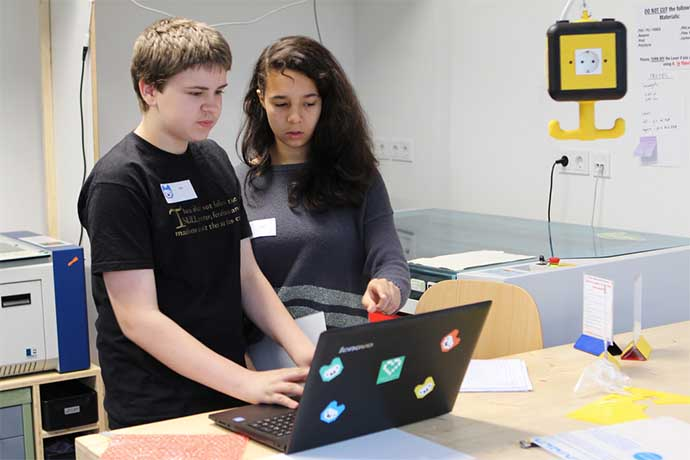Hour of Code and EU Code Week are events designed to introduce kids, young adults, and others to programming and computer science.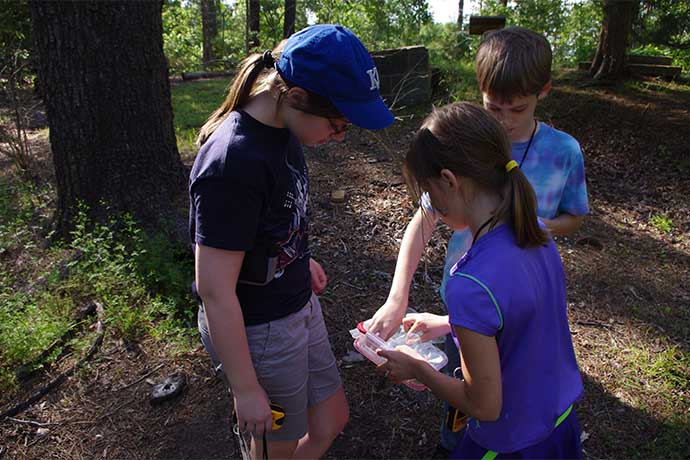Real life treasure hunts are a way to get outdoors, learn map skills, and have fun finding hidden caches near you.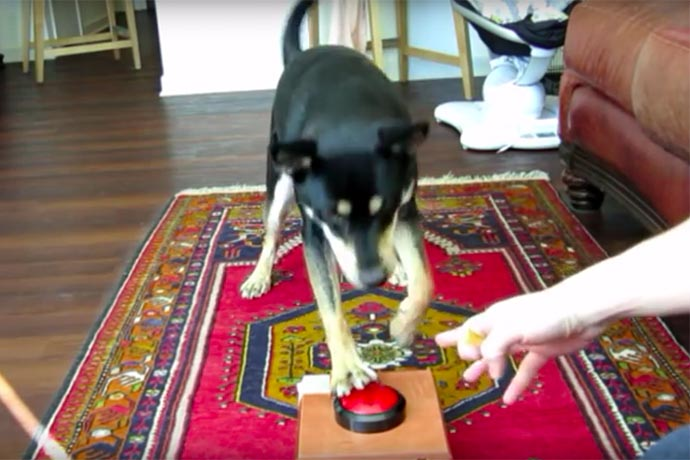A trainable puppy plus treats plus technology equals a dog that can send selfies. Here's how.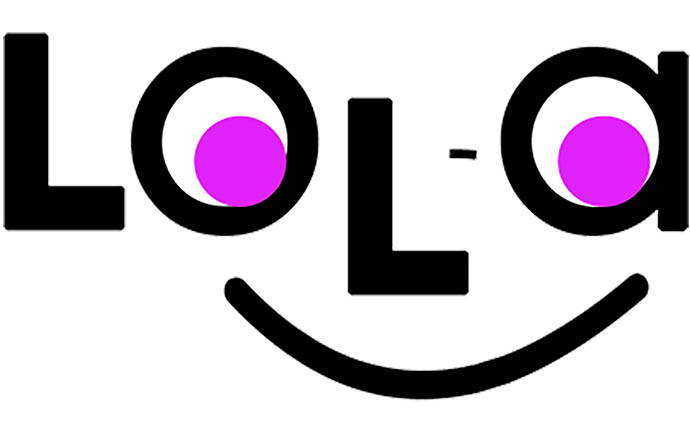An app to help kids remember important stuff like feed your pets, brush your teeth, and smile.These books include lots of great projects to work on by yourself or with others, from Scratch and Minecraft to fun maker space projects.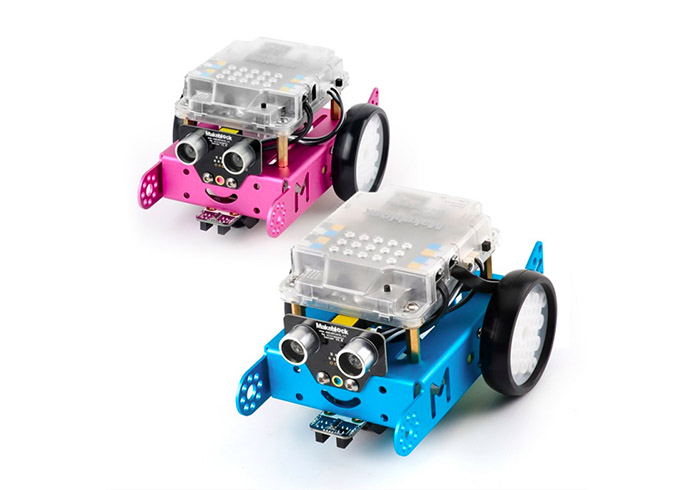The mBot robotics kit is an excellent comparatively low-cost way to begin working with robots.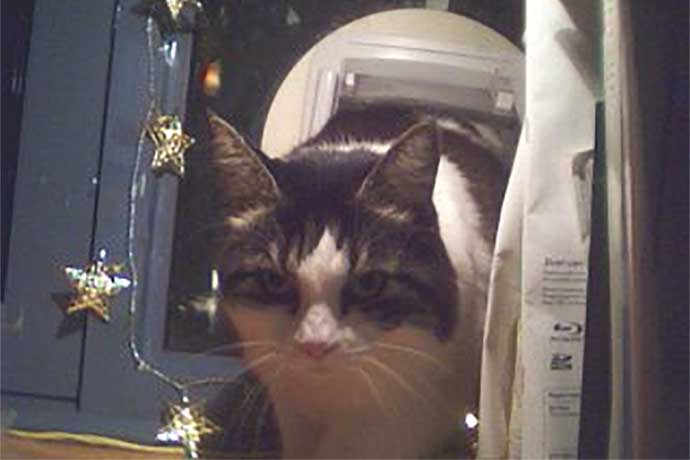There are maybe a bazillion Raspberry Pi projects online. Here are really fun projects plus links to find more.The Wayback Machine lets you travel back in time to see old websites. Plus the Internet Archive has thousands of vintage games, software, books, and more.Eating dog food doesn't sound like much fun but it's an important part of creating software.The ability to identify patterns, decompose large problems into small parts, develop algorithms to solve problems, and generalize to find solutions.To celebrate this wonderful time of the year, let’s create some holiday music using Sonic Pi on our Raspberry Pi.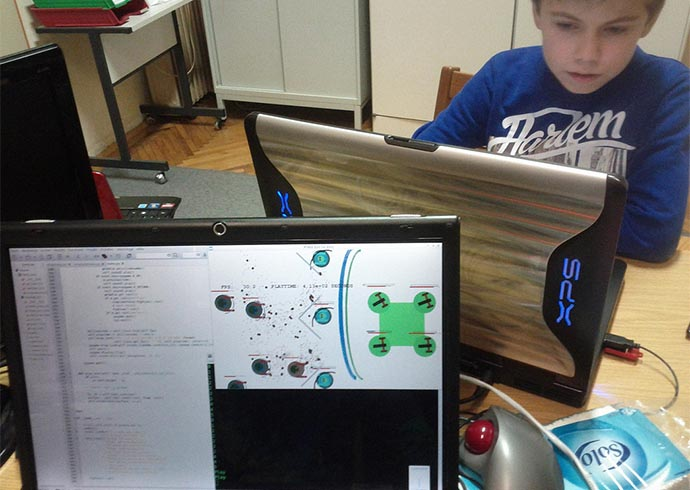This project shows how to use the pygame code library to move simple animations with the Python programming language.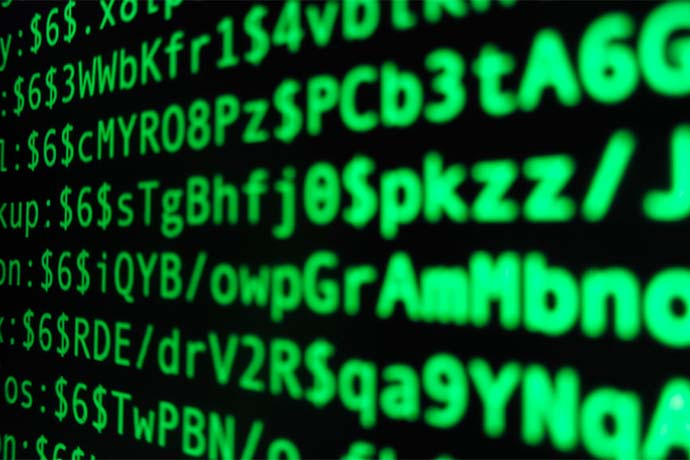This project, shows you how to create your own random password generator in the C# programming language.This project teaches you about binary numbers and how to translate them to decimal numbers we recognize.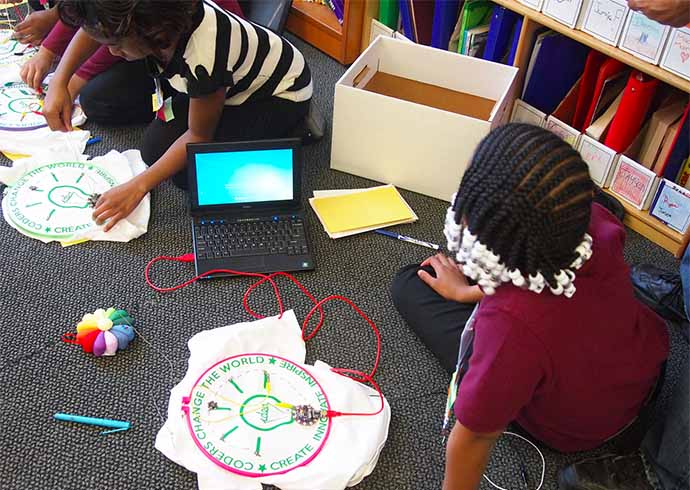These projects mix science and technology in interesting ways. Sewing and electronics, for example, is a different way to learn about electronics.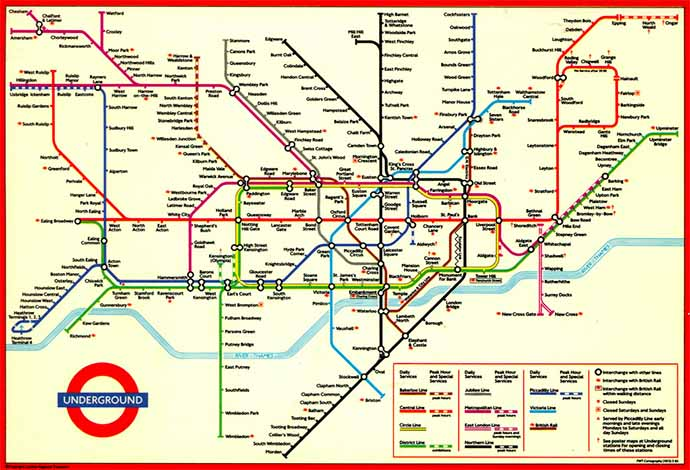Links from the bottom of all the December 2016 articles, collected in one place for you to print, share, or bookmark.Interesting stories about computer science, software programming, and technology for December 2016.What sounds like a country western dance actually is an efficient way to sort large sets of data randomly.